# ML Aggarwal Class 8 Solutions for ICSE Maths Chapter 14 Constructions of Quadrilaterals Ex 14.1

## ML Aggarwal Class 8 Solutions for ICSE Maths Chapter 14 Constructions of Quadrilaterals Ex 14.1

Question 1.
Construct a quadrilateral PQRS where PQ = 4.5 cm, QR = 6 cm, RS = 5.5 cm, PS = 5 cm and PR = 6.5 cm.
Solution: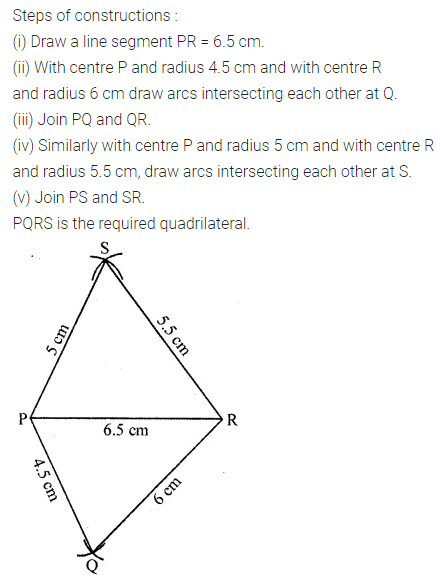Question 2.
Construct a quadrilateral ABCD in which AB = 3·5 cm, BC = 5 cm, CD = 5·6 cm, DA = 4 cm and BD = 5·4 cm
Solution: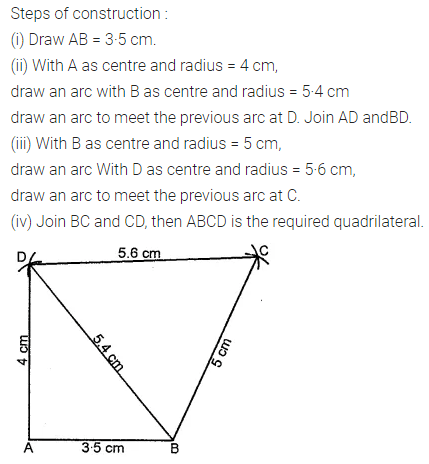Question 3.
Construct a quadrilateral PQRS in which PQ = 3 cm, QR = 2·5 cm, PS = 3·5 cm, PR = 4 cm and QS = 5 cm.
Solution:Question 4.
Construct a quadrilateral ABCD such that BC = 5 cm, AD = 5.5 cm, CD = 4.5 cm, AC = 7 cm, and BC = 5.5 cm.
Solution: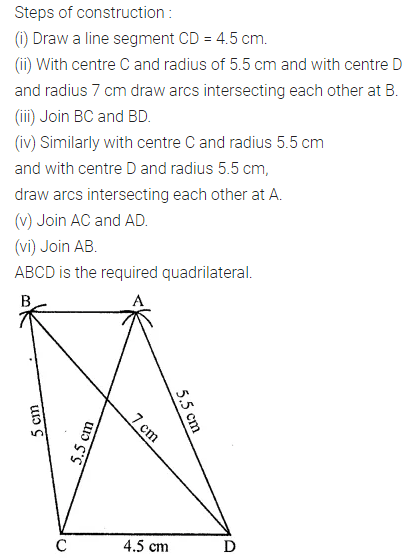Question 5.
Construct a quadrilateral ABCD given that BC = 6 cm, CD = 4 cm, ∠B = 45°, ∠C = 90° and ∠D = 120°.
Solution: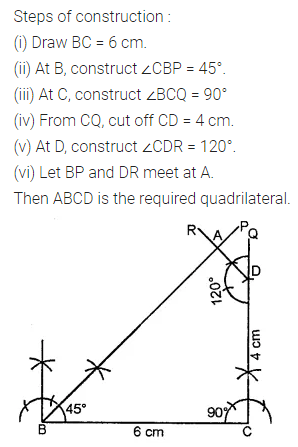Question 6.
Construct a quadrilateral PQRS where PQ = 4 cm, QR = 6 cm, ∠P = 60°, ∠Q = 90° and ∠R = 120°.
Solution:Question 7.
Construct a quadrilateral ABCD such that AB = 5 cm, BC = 4·2 cm, AD = 3·5 cm, ∠A = 90°, and ∠B = 60°
Solution: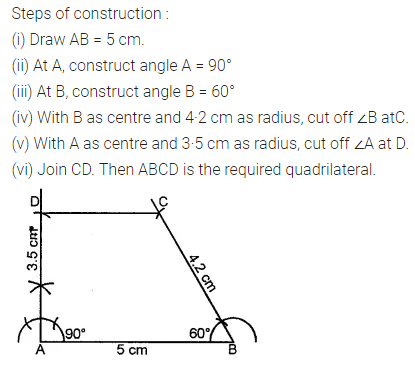Question 8.
Cosntruct a quadrilateral PQRS where PQ = 4 cm, QR = 5 cm, RS = 4.5 cm, ∠Q = 60° and ∠R = 90°.
Solution: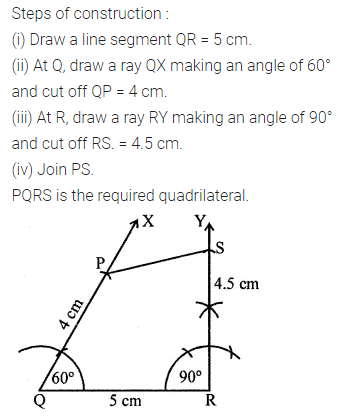Question 9.
Construct a quadrilateral BEST where BE = 3.8 cm, ES = 3.4 cm, ST = 4.5 cm, TB = 5 cm and ∠E = 80°.
Solution: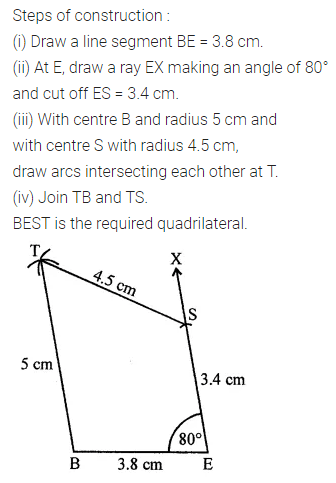Question 10.
Construct a quadrilateral ABCD where AB = 4·5 cm, BC = 4 cm, CD = 3·9 cm, AD = 3·2 cm and ∠B = 60°.
Solution: